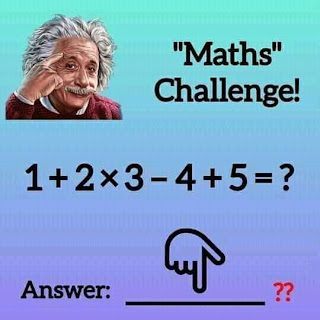0

# What's 1+2×3-4+5=? Math Quiz answerWhat's 1+2×3-4+5=? Math Quiz answer
This tricky math quiz is Trending online today. Many people failed it. I will solve and give you the correct answer.

## What's 1+2×3-4+5=? Math challenge answer

The correct answer to  'What's 1+2×3-4+5=? Math Quiz answer' would be 8. I will solve it in detail below by applying BODMAS.

The secret to get all your answers correct when solving a Quiz like the one above is to always Apply BODMAS. So, I will solve this '1+2×3-4+5=?' using BODMAS. Also note that you don't have to add brackets again before solving because you will indirectly create another Quiz. Many people did this when proving their wrong answer on Facebook.

Be sure to share with friends.

Let's get started

1+2×3-4+5=? The first thing is to multiply.

Implied 1+6- 4 + 5 = ?

12- 4 = ?

8 = ?

Hence, the correct answer is 8.

Conclusion

If you have different opinions, Comment.